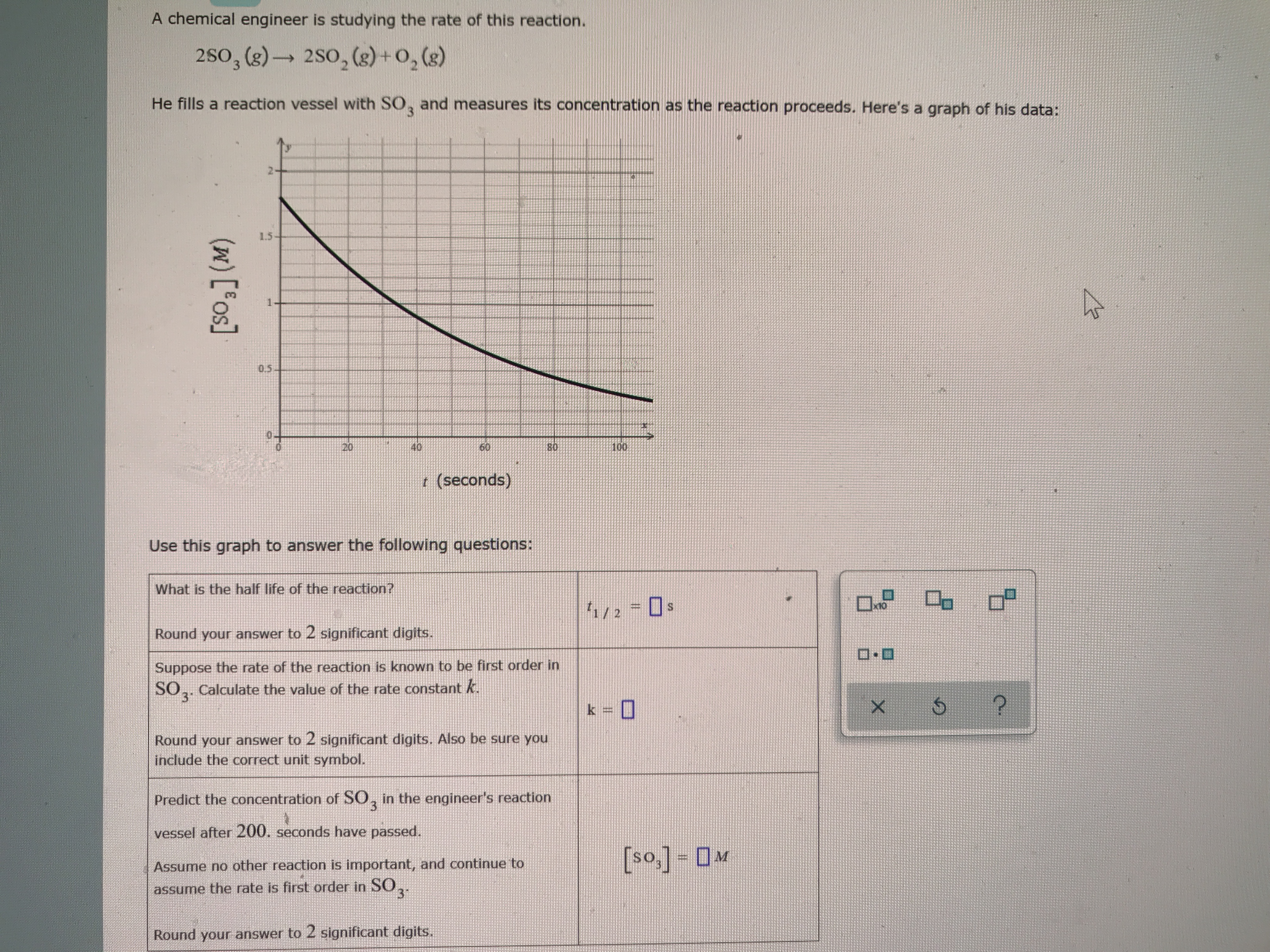# A chemical engineer is studying the rate of this reaction. 2 SO3(g) → 2 SO2(g) + O2(g) He fills a reaction vessel with SO3 and measure its concentration as the reaction proceeds. Here's a graph of his data: What is the half-life of this reaction? Suppose the rate of reaction is known to be first order in SO3. Calculate the value of rate constant k. Predict the concentration of SO3 in the engineer's reaction vessel after 200 seconds have passed.